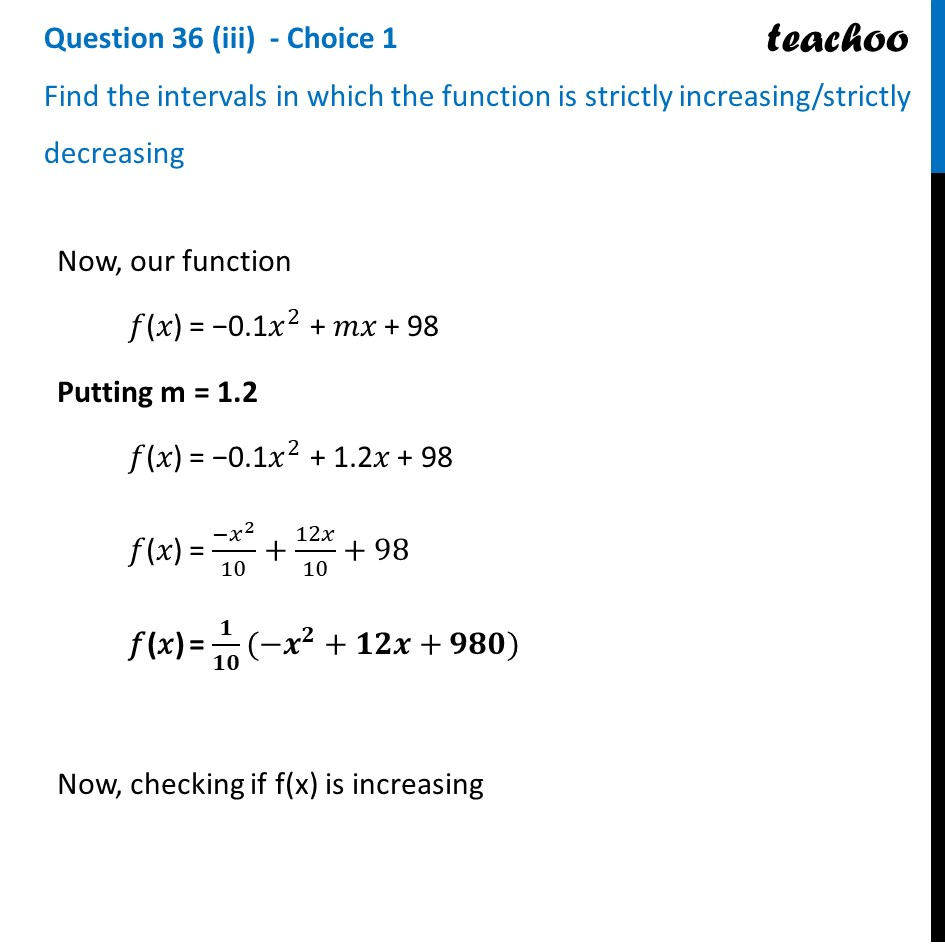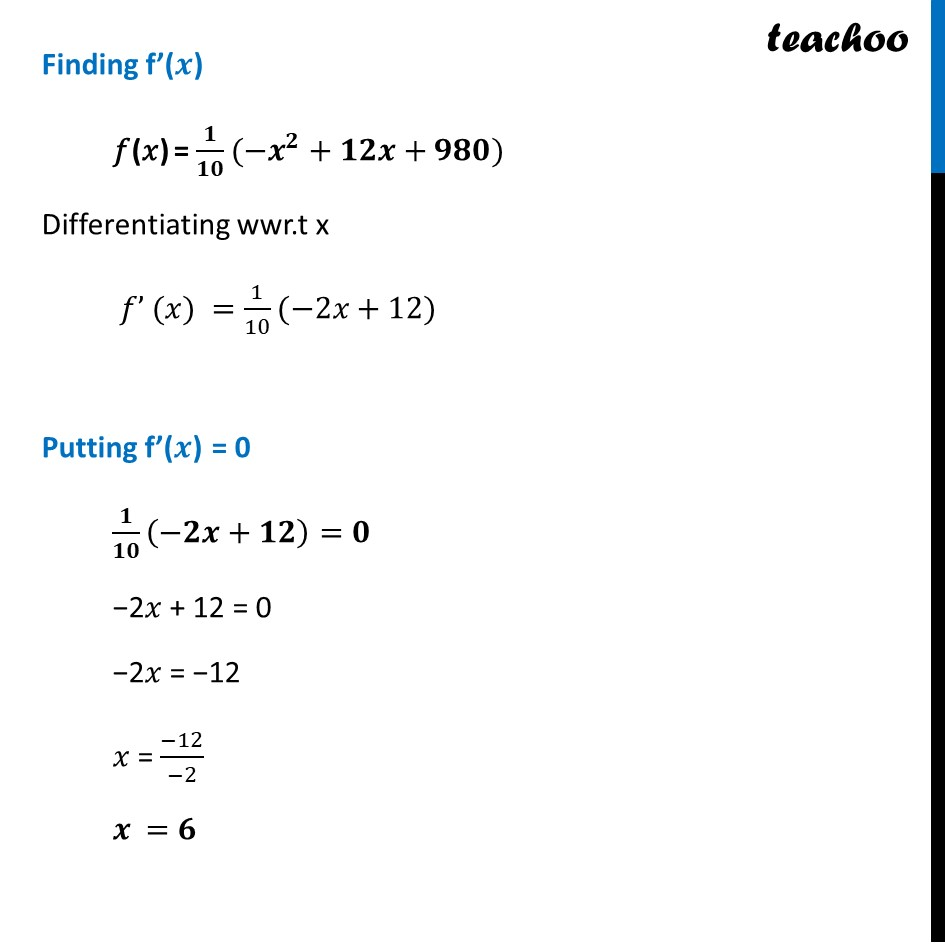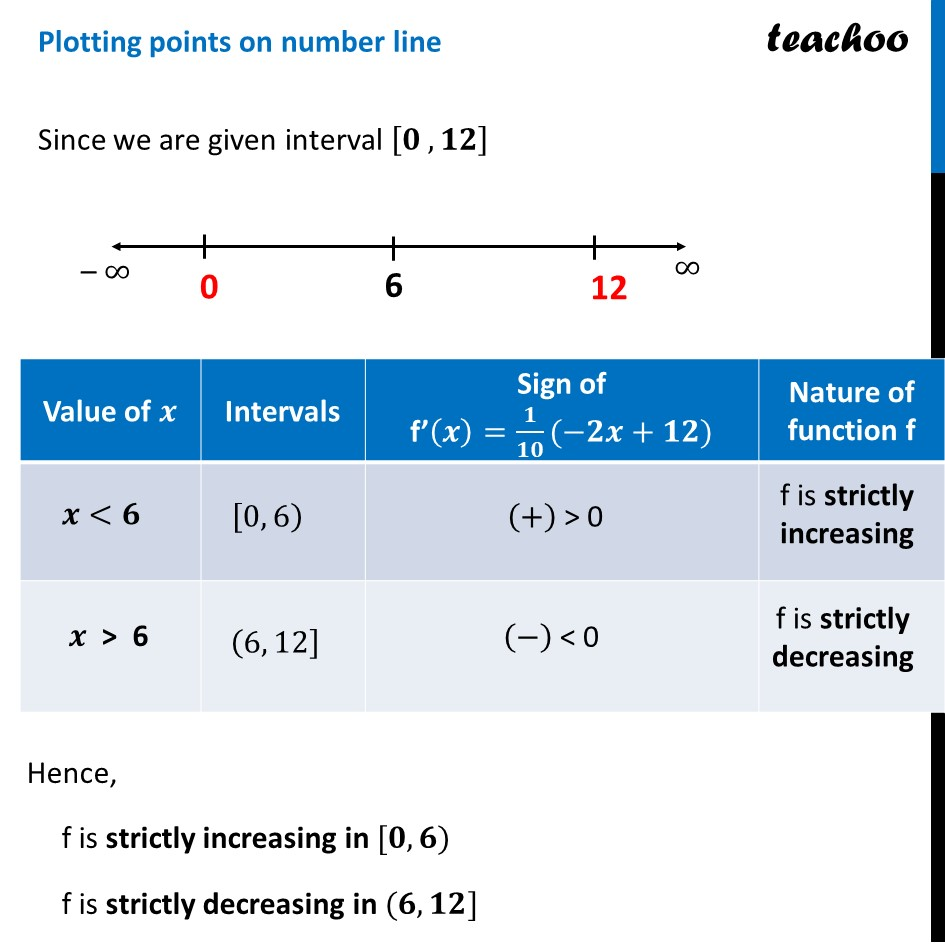CBSE Class 12 Sample Paper for 2023 Boards

Class 12
Solutions of Sample Papers and Past Year Papers - for Class 12 Boards

## Find the intervals in which the function is strictly increasing/strictly decreasingLearn in your speed, with individual attention - Teachoo Maths 1-on-1 Class

### Transcript

Question 36 (iii) - Choice 1 Find the intervals in which the function is strictly increasing/strictly decreasingNow, our function 𝑓(𝑥) = −0.1𝑥^2 + 𝑚𝑥 + 98 Putting m = 1.2 𝑓(𝑥) = −0.1𝑥^2 + 1.2𝑥 + 98 𝑓(𝑥) = 〖−𝑥〗^2/10+12𝑥/10+98 𝑓(𝑥) = 𝟏/𝟏𝟎(−𝒙^𝟐+𝟏𝟐𝒙+𝟗𝟖𝟎) Now, checking if f(x) is increasing Finding f’(𝒙) 𝑓(𝑥) = 𝟏/𝟏𝟎(−𝒙^𝟐+𝟏𝟐𝒙+𝟗𝟖𝟎) Differentiating wwr.t x 𝑓’ (𝑥) =1/10(−2𝑥+12) Putting f’(𝒙) = 0 𝟏/𝟏𝟎 (−𝟐𝒙+𝟏𝟐)=𝟎 −2𝑥 + 12 = 0 −2𝑥 = −12 𝑥 = (−12)/(−2) 𝒙 =𝟔 Plotting points on number line Since we are given interval [𝟎 , 𝟏𝟐] Hence, f is strictly increasing in [𝟎, 𝟔) f is strictly decreasing in (𝟔,𝟏𝟐]